NEET  >  Semiconductors: Properties & Types

# Semiconductors: Properties & Types - Notes | Study Physics Class 12 - NEET

 Table of contentsProperties of SemiconductorsTypes of Semiconductor1 Crore+ students have signed up on EduRev. Have you?

Semiconductors are the materials that have a conductivity between conductors (generally metals) and non-conductors or insulators (such as ceramics). Semiconductors can be compounds such as gallium arsenide or pure elements, such as germanium or silicon. Physics explains the theories, properties and mathematical approach governing semiconductors.

Properties of Semiconductors

• Semiconducting elements are tetra-valent i.e. there are four electrons in their outermost orbit.
• Their lattice is face centered cubic (F.C.C.)
• The number of electrons or cotters is given by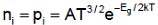i.e. on increasing temperature, the number of current carriers increases.
• There are uncharged.
• Semiconductor acts like an insulator at Zero Kelvin. On increasing the temperature, it works as a conductor.
• Due to their exceptional electrical properties, semiconductors can be modified by doping to make semiconductor devices suitable for energy conversion, switches, and amplifiers.
• Lesser power losses.
• Semiconductors are smaller in size and possess less weight.
• Their resistivity is higher than conductors but lesser than insulators.
• The resistance of semiconductor materials decreases with the increase in temperature and vice-versa.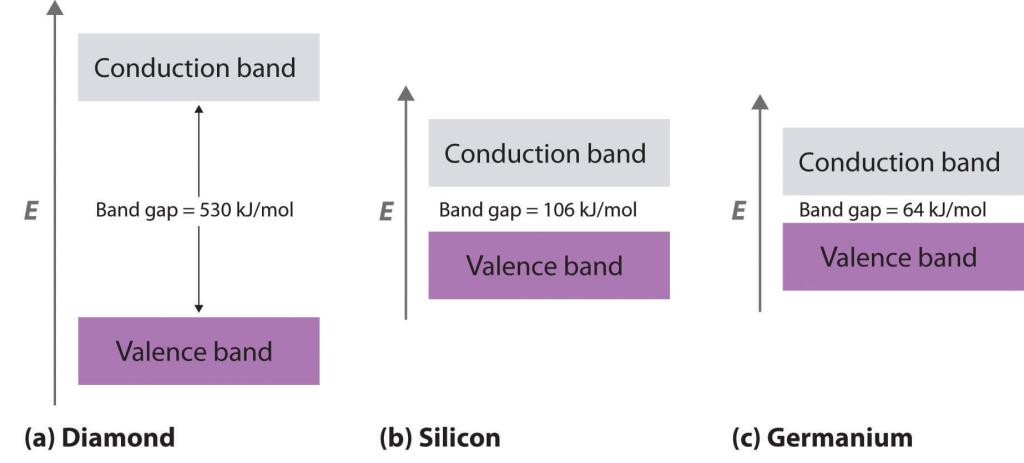Example of different semi-conductors

• Holes or cotters
(i) The deficiency of electrons in covalent band formation in the valence band in defined as hole or cotter,
(ii) These are positively charged. The value of positive charge on them is equal to the electron charge.
(iii)  Their effective mass is less than that of electrons.
(iv)  In an external electric field, holes move in a direction opposite to that of electrons i,e. they move from positive to negative terminal.
(v)  They contribute to current flow.
(vi)  Holes are produced when covalent bonds in valence band break.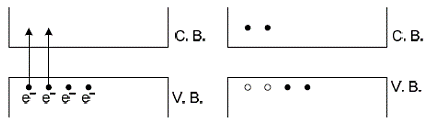Transfer of electrons from valence band to conduction band

Types of Semiconductor

Semiconductors can be classified as:

• Intrinsic Semiconductor
• Extrinsic Semiconductor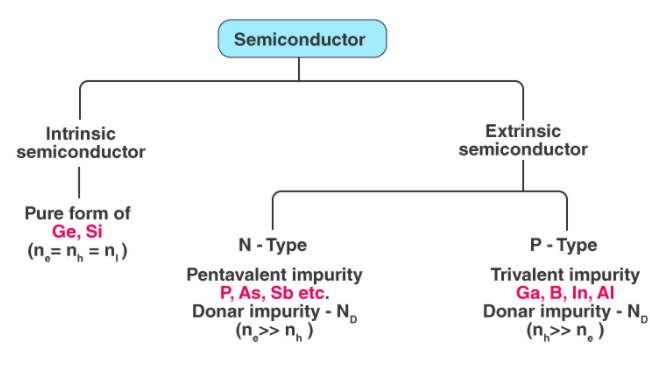Intrinsic Semiconductor

• An intrinsic type of semiconductor material is made to be very pure chemically. It is made up of only a single type of element.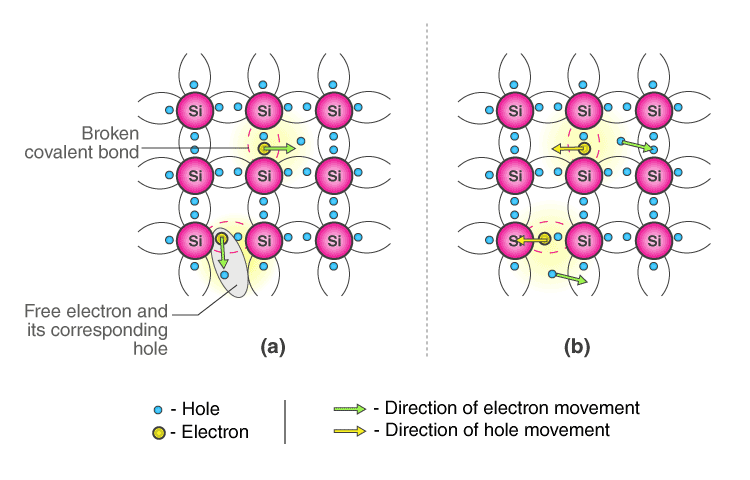Conduction Mechanism in Case of Intrinsic Semiconductors (a) In absence of electric field (b) In presence of electric Field

• Germanium (Ge) and Silicon (Si) are the most common type of intrinsic semiconductor elements. They have four valence electrons (tetravalent). They are bound to the atom by covalent bond at absolute zero temperature.
• When the temperature rises, due to collisions, few electrons are unbounded and become free to move through the lattice, thus creating an absence in its original position (hole). These free electrons and holes contribute to the conduction of electricity in the semiconductor. The negative and positive charge carriers are equal in number.
• The thermal energy is capable of ionizing a few atoms in the lattice, and hence their conductivity is less.

➢ The Lattice of Pure Silicon Semiconductor at Different Temperatures

• At absolute zero Kelvin temperature: At this temperature, the covalent bonds are very strong and there are no free electrons and the semiconductor behaves as a perfect insulator.
• Above absolute temperature: With the increase in temperature few valence electrons jump into the conduction band and hence it behaves like a poor conductor.

➢ Energy Band Diagram of Intrinsic Semiconductor

• The energy band diagram of an intrinsic semiconductor is shown below: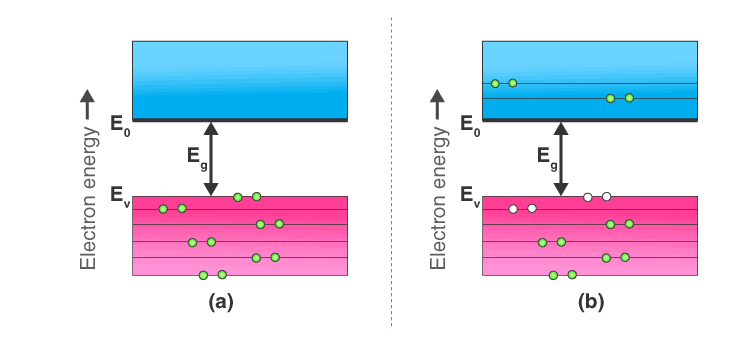(a) Intrinsic Semiconductor at T = 0 Kelvin, behaves like an insulator (b) At t>0, four thermally generated electron pairs
• In intrinsic semiconductors, current flows due to the motion of free electrons as well as holes. The total current is the sum of the electron current Ie due to thermally generated electrons and the hole current Ih
Total Current (I) = Ie + Ih
• For an intrinsic semiconductor, at finite temperature, the probability of electrons to exist in conduction band decreases exponentially with increasing bandgap (Eg)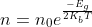Where,
Eg = Energy bandgap
Kb = Boltzmann’s constants

Extrinsic Semiconductor

• The conductivity of semiconductors can be greatly improved by introducing a small number of suitable replacement atoms called impurities.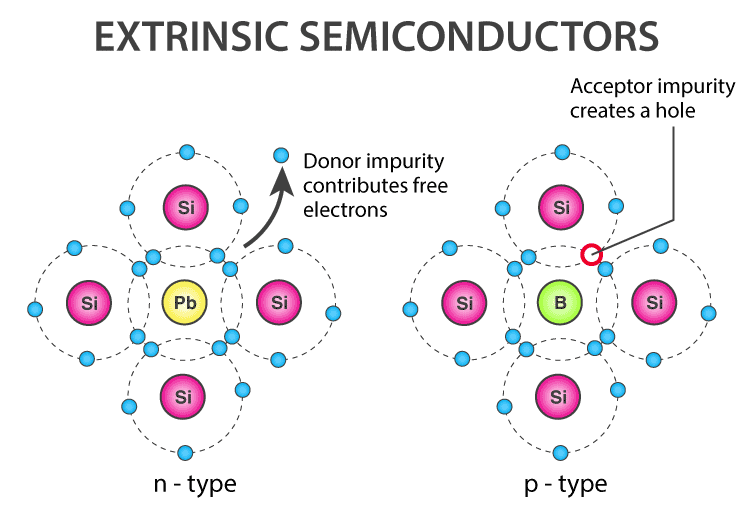• The process of adding impurity atoms to the pure semiconductor is called doping
• Usually, only 1 atom in 107 is replaced by a dopant atom in the doped semiconductor.
• An extrinsic semiconductor can be further classified into:
(i) N-type Semiconductor
(ii) P-type Semiconductor

Table: Difference between intrinsic and extrinsic semiconductors

 S.No. Intrinsic semiconductors Extrinsic semiconductors 1. Pure Ge or Si is known as intrinsic semiconductor The semiconductor, resulting from mixing impurity in it, is known as extrinsic semiconductors. 2. Their conductivity is low (because only one electron in 109 contribute) Their conductivity is high 3. The number of free electrons (m in conduction band Is equal to the number of holes pi in valence band.) In these ni ≠pi 4. These are not practically used These are practically used 5. In these the energy gap is very small In these the energy gap is more than that in pure semiconductors. 6. In these the Fermi energy level lies in the middle of valence band and conduction In these the Fermi level shifts towards valence or conduction energy bands.

➢ Properties of Extrinsic Semiconductors

• At absolute zero temperature (0 K) there are no free electrons in them.
• At room temperature, the electron-hole pair in sufficient number are produced.
• Electric conduction takes place via both electrons and holes.
• The drift velocities of electrons and holes are different.
• The drift velocity of electrons (Vdn) is greater than that of holes (Vdp).
• The total current is I = In + Jp.
• In connecting wires the current flows only via electrons.
• The current density is given by: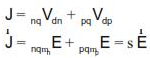Where Vdn = drift velocity of electrons
μn = mobility of electrons
Vdp = drift velocity of holes
μp = mobility of holes
• The electric conductivity is given by s = nq(mn + mp).
• Mobility of electron mn = Vdn / E.
• Mobility of holes mp = Vdp/E.
• At room temperature sGe > sSi because nGe > nSi
where nGe = 2.5' 1013/cm3 and nSi = 1.4' 1010 /cm3.
The document Semiconductors: Properties & Types - Notes | Study Physics Class 12 - NEET is a part of the NEET Course Physics Class 12.
All you need of NEET at this link: NEET

## Physics Class 12

157 videos|452 docs|213 tests
 Use Code STAYHOME200 and get INR 200 additional OFF

## Physics Class 12

157 videos|452 docs|213 tests

### How to Prepare for NEET

Read our guide to prepare for NEET which is created by Toppers & the best Teachers

Track your progress, build streaks, highlight & save important lessons and more!

,

,

,

,

,

,

,

,

,

,

,

,

,

,

,

,

,

,

,

,

,

;